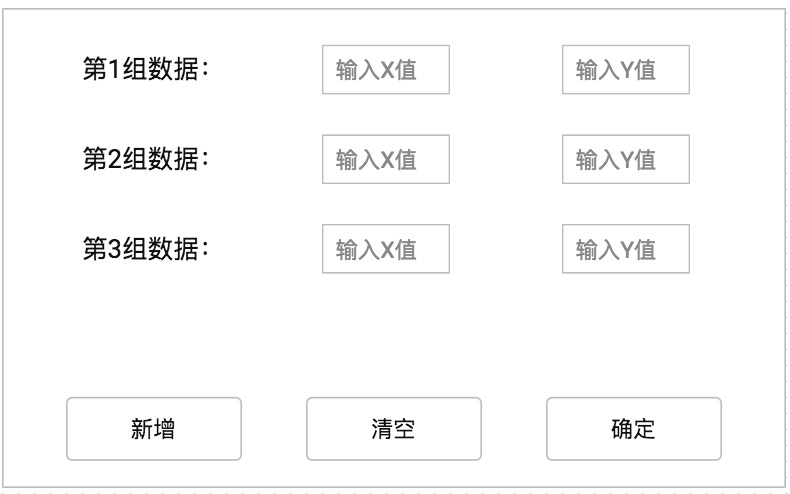from tkinter import *
root = Tk()
root.title("元素周期表")
def cal():
master = Tk()
v1=StringVar()
v2=StringVar()
v3=StringVar()
frame = Frame(master)
Entry(frame,width=10,textvariable=v1).grid(row = 0, column = 1)
Entry(frame,width=10,textvariable=v2).grid(row = 1, column = 1)
Entry(frame,width=10,textvariable=v3).grid(row = 2, column = 1)
Button(frame,text="数1").grid(row=0,column=0)
Button(frame,text="数2").grid(row=1,column=0)
Button(frame,text="数3").grid(row=2,column=0)

Button(root,text="选择",command=cal).grid(row=2,column=1)
Button(root,text="输入一个数",command=cal).grid(row=2,column=1)
Button(root,text="输入另一个数",command=cal).grid(row=2,column=2)

mainloop()

0

3个回答

`````` from tkinter import *

root = Tk()
root.title("元素周期表")

def cal(type):
master = Tk()
v1 = StringVar()
v2 = StringVar()
v3 = StringVar()
frame = Frame(master)

Entry(frame, width=10, textvariable=v1).grid(row=0, column=1)
Button(frame, text="数1").grid(row=0, column=0)

if (type == 1): return;
Entry(frame, width=10, textvariable=v2).grid(row=1, column=1)
Button(frame, text="数2").grid(row=1, column=0)

if (type == 2): return;
Entry(frame, width=10, textvariable=v3).grid(row=2, column=1)
Button(frame, text="数3").grid(row=2, column=0)

Button(root, text="选择", command=cal).grid(row=2, column=1)
Button(root, text="输入一个数", command=lambda :cal(1)).grid(row=2, column=1)
Button(root, text="输入另一个数", command=lambda :cal(2)).grid(row=2, column=2)

mainloop()
``````
0fanshinee 回复caozhy: 您好，没有 办法传图，您看下我回复的那个fanshinee 老师您好，使用tkinter中的button和enry按钮，增加输入框（通过），这些输入框怎么能够有序的排列，并且按照某种运算（例如加法）运算？fanshinee 您好，非常感谢您的回复，可是我刚刚测试了下，您这个每次点击一下都会出现一个新的小窗口，我希望每次都出现在一个小窗口。

00js选择一个选项 跳出另一个选项 跳出一个输入框

js 当在文本框中输入文字时对应的多选项同时勾选中

HTML中表单如何给输入框绑定待选项

js 选择一个选择框选项,影响另一个input才可以输入
1.&amp;lt;select&amp;gt;标签里要添加onchange事件；

rn 针对EasyUI 1.5.1 版本在6791行下，添加如下代码：rnrn//datebox单击文本框出现日期选择 startrnif (\$(_4ed).hasClass(&quot;datebox-f&quot;)) {rn _4f2.click(function() {rn _4f4.click();rn });rn}rn// endrnrn添加完后上下文代码如下：rnrnvar...
1、select，弹出相应文本框说明：当select选中值改为else，其值为“-1”时，显示一个自定义的输入框，若要将自定义的项加入到select的数据项中，需在后台将自定义项存入对应的数据表中，这样，在刷新后，下一次访问这个select时，该自定义项就会出出现在select的选项中。项目的技术成果发布表单中的就有该功能的应用。js：n function chg(obj)n {n

【android开发】弹出选择框

jquery点击输入框时弹出div层复选框勾选输入的内容

Jsp部分：rnrn 其它rnrnrnrnJS部分：rnfunction energyTypeCheck (chk) {rnvar Checkbox5 = document.getElementById('Checkbox5');rnif (chk.checked) {rnCheckbox5.style.display='inline-block';rn}else {rnCheckbox5.sty

Python tkinter实现先弹出选择框后弹出输入框，并得到输入框的值
Python 自带的GUI tkinter实现界面编写有点难，这次我遇到的问题如下所示：当我一开始进入系统时弹出如下选择框，我点击确定即可弹出输入框，可当我输入好相应信息之后点击确定，却拿不到我在输入框输入的值。这个情况的具体代码如下：import tkinter as tknfrom tkinter import messageboxnndef on_click():n global U...
layui当点击文本框时弹出选择框，显示选择内容

js 点击input弹出选择框
[size=large][color=red]定义选择框html[/color][/size]rn[code=&quot;js&quot;]rnvar str = &quot;&quot;;rn document.writeln(&quot;&quot;);rn str += &quot;\u65e5&quot;;rn for (i = 1; i

JS脚本根据select选择的值弹出输出框

js插件-弹出层-选择框-输入框

nnJsn function submitError() {n var HTML = &quot;&quot;;n HTML += &quot;&amp;lt;input type='hidden' name='ecp_type' value='170'/&amp;gt;&quot;;n HTML += &quot;&amp;lt;div&amp;gt;&amp;lt;div style='float:left;'&amp;gt;异常件类型:&amp;amp;...
（四）html输入框提示和输入选择
required的使用：nn n 提交nnlabel的使用：nn这里写js代码nnn姓名：
android弹出消息框、输入框、选择框、日期选择

Android开发使输入框点击弹出日期选择对话框的方法
Android开发使输入框点击弹出日期选择对话框的方法rn rn转载▼        非常简单直接上代码：rnrnrn获得显示的控件,赋值调用就行  很简单 效果图 上代码↓↓↓↓↓↓↓rnrnrnrnrnrnrnrn        final EditText et1=(EditText)findViewById(R.id.editText1); //获取点击显示日期选择的的控件rn

&amp;lt;%--下拉框与选项的二级联动--%&amp;gt;n&amp;lt;select id=&quot;reviewType&quot; class=&quot;form-control&quot; name=&quot;reviewValue&quot; onchange=&quot;requestReviewType()&quot;&amp;gt;n &amp;lt;option value=&quot;0&quot; label=&quot;--请选择--&quot; hidden=&

Android输入框下拉列表

iOS开发——ActionSheet的使用与弹出选择对话框